# Grafix Users Guide

This is a quick look at Grafix, our tool for exploring matrices via 3-D computer graphics.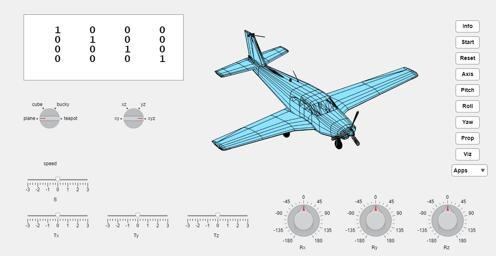### Contents

#### Matrices

The word matrix comes from the Latin word mater, which means mother or origin. Matrices are like a mother to much of modern applied mathematics and computational science.

Grafix is a study of the matrices that describe rotation, translation and scaling of objects moving in three-dimensional space. These matrices are the building blocks of today's computer graphics and are essential to all popular video games, to all CAD (Computer Aided Design) packages, to CGI (Computer Graphics Imagery) in films, and to MATLAB's Handle Graphics.

MATLAB is short for Matrix Laboratory. Matrices are also the foundation of MATLAB and of MathWorks.

The homogeneous coordinates system used in modern computer graphics makes it possible to describe rotations, translations and many other operations with 4-by-4 matrices. These matrices operate on vectors with the position of an object in the first three components and, for now, a one as the fourth component, e.g. [x,y,z,1]',

Matrices are always shown in a panel on the Grafix window. Here is an animation of one important example. Do you see the pattern in the evolving elements of this matrix? How are they related to each other? How long before they repeat?#### Axis

Here is the coordinate system used by view(3), MATLAB's default projection of our three-dimensional physical world onto the two-dimensional computer display. The positive x-axis goes up and to the right on the screen, the positive y-axis goes up and to the left, and the positive z-axis goes straight up.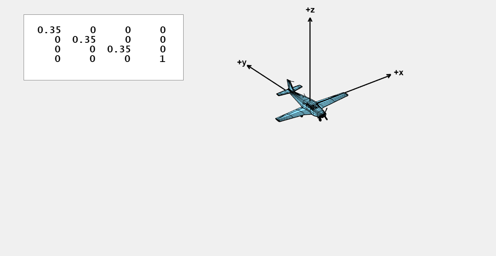#### Rx

The three knobs at the lower right of the Grafix window allow you to vary the angles of Rx, Ry and Rz. Here are snapshots of the resulting motion. The Rx knob produces rotation about the x-axis, leaving x unchanged while rotating y and z.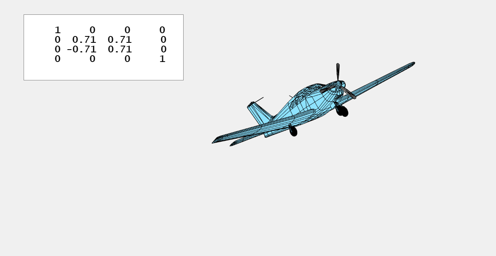#### Ry

Ry, rotation about the y-axis, leaves y unchanged while rotating x and z.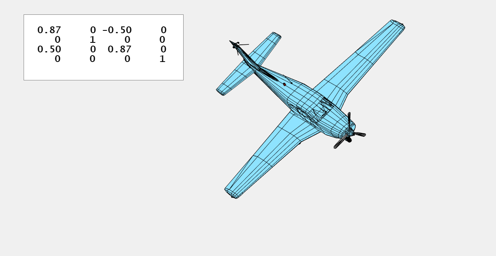#### Rz

And, Rz, rotation about the z-axis, leaves z unchanged while rotating x and y.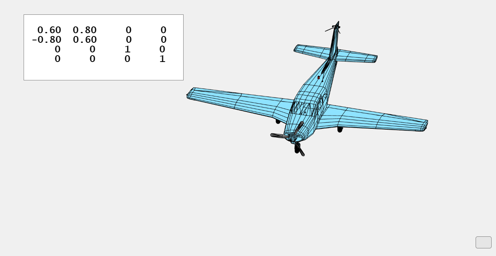#### Tx

The three sliders at the lower left of the Grafix window control matrices with values in the fourth column. Multiplying a vector by Tx, which has a nonzero element in the first row, produces a horizontal movement in the direction of the x-axis.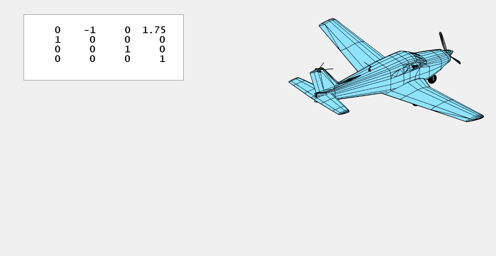#### Ty

Ty, with a nonzero in the second row, is translation in the direction of the y-axis.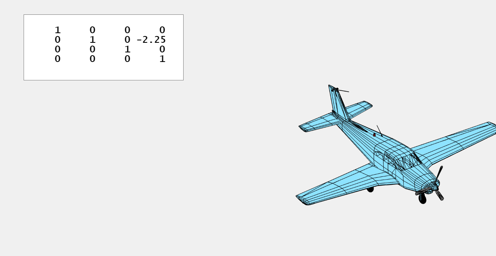#### Tz

And, Tz is translation in the z direction.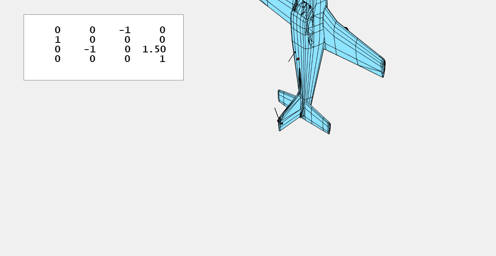#### S

The fourth slider controls S, a diagonal matrix with a single scaling factor for all three axes.

The use of matrix multiplication allows translations and scaling to be combined with rotations and other operations in a uniform way. The arithmetic units on today's Graphics Processing Units, GPUs, are designed to do 4-by-4 matrix multiplications at speeds hundreds of times faster than general purpose Central Processing Units, CPUs.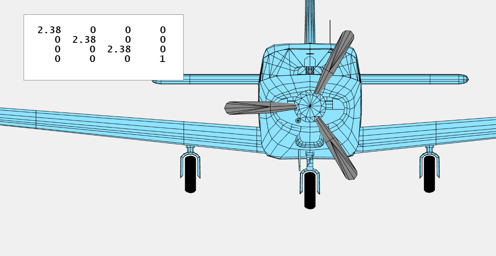#### Switches

The switch on the left provides other objects that we will introduce later. The switch on the right provides different views of three dimensions. The yz plane view is shown here.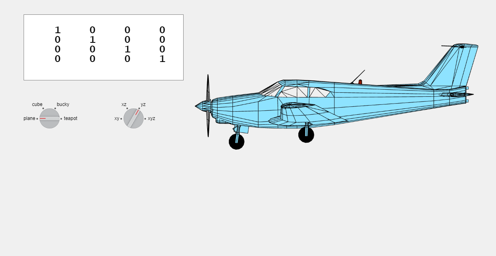#### Pitch, Roll, Yaw

Pitch, roll and yaw are often used to describe the motion of aircraft and spacecraft. These terms are closely related to the rotations Rx, Ry and Rz. The Pitch button animates the Rx knob. Here is a snapshot near one extreme of the resulting motion.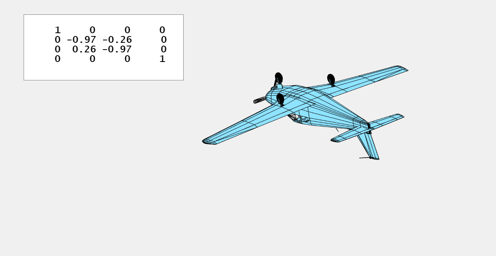#### Start, Reset, Viz

The Start button restarts Grafix. The Reset button resets all knobs and sliders.

The Viz button turns off the display of buttons, knobs, sliders, and switches. A small button, emphasized here with color, but usually barely visible, turns the display back on.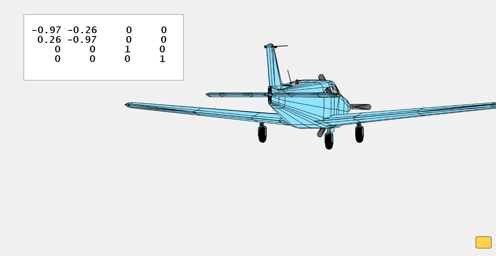#### Apps/taxi

Grafix is programable, in a primitive sort of way. Apps are Grafix programs. Taxi is a small app. This is a snapshot.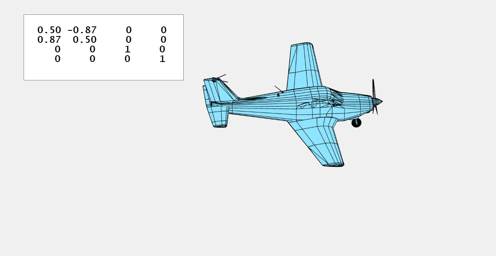#### Apps/takeoff

When the takeoff app first appeared, I thought it was a bug in the code for Grafix.

You can see the takeoff program by entering type takeoff at the MATLAB command prompt. You can also edit takeoff.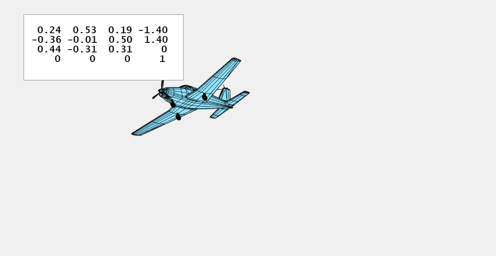#### Prop

One of my favorite animations employs the rotations from the Matrices section to drive the propeller alone.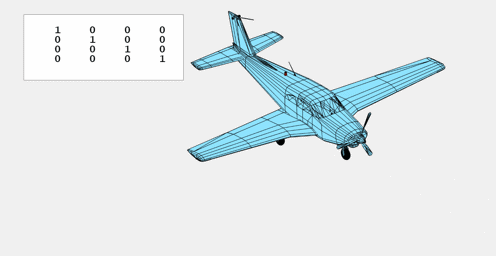#### Info

The info button is a link to this User's Guide.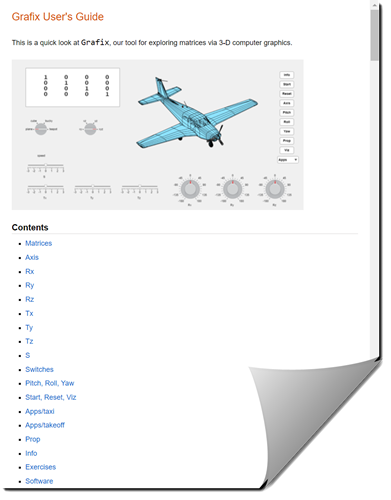#### Exercises

• What color is the beacon on top of the plane?
• Demonstrate how matrix-vector multiplication, M*v, of a position vector, v = [x,y,z,1]', by a 4-by-4 matrix M, achieves a rotation, translation or scaling.
• Describe the evolution of the matrix values in the Matrices and prop animations.
• What angles are involved in the snapshots shown for Rx, Ry and Rz?
• Why is the plane in the Pitch animation upside down?
• What angles and what matrices characterize the extremes of the Pitch animation?
• Write another app like taxi and takeoff.

#### Software

The MATLAB code for Grafix is available here.

Published with MATLAB® R2023a

|